## CYLINDERIZE

 Applies a cylinder distortion to an image so that the image is wrapped about the cylinder

### EXAMPLES

 No Pitch Original ImageArguments: -m vertical Arguments: -m vertical -l 56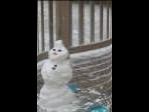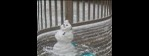Arguments: -m horizontal Arguments: -m horizontal -l 75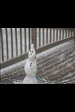Arguments: -m vertical -v background -b red Arguments: -m vertical -r 75Rotation Animation Arguments: -m vertical -w 100 -a # (where # varies from 0 to 360 in 30 degree increments)Rotation Animation Arguments: -m vertical -w 50 -p 25 -a # (where # varies from 0 to 360 in 30 degree increments)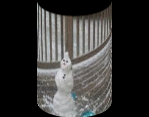With 20 Degree Pitch Original ImageArguments: -m vertical -p 20 Arguments: -m vertical -l 56 -p 20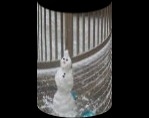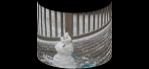Arguments: -m horizontal -p 20 Arguments: -m horizontal -l 75 -p 20Arguments: -m vertical -v background -b red -p 20 Arguments: -m vertical -r 75 -p 20Arguments: -m vertical -p 20 -t Arguments: -m vertical -v background -b none -p 20 -t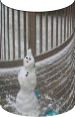With 45 Degree Pitch Original ImageArguments: -m vertical -p 45 Arguments: -m horizontal -p 20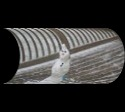Overlay Example 1 Input Image (Source Image)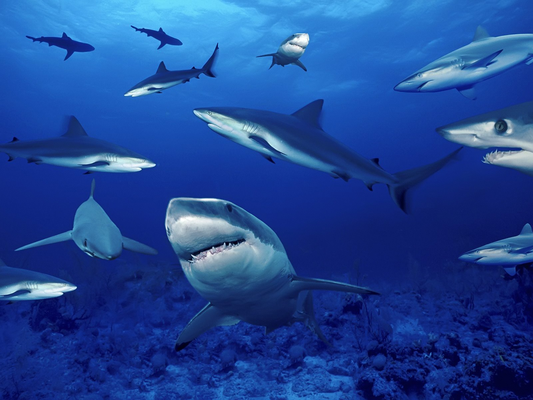Background Image (Source Image) Background Image (Source Image)Arguments: -m vertical -r 73 -l 120 -w 90 -p 5 -n 94 -e 2 -a 100 -v background -b none -f none -o +24+10 Arguments: -m vertical -r 73 -l 120 -w 90 -p 5 -n 94 -e 2 -a -100 -v background -b none -f none -o -26+10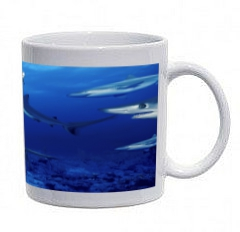Arguments: -m vertical -r 73 -l 120 -w 90 -p 5 -n 94 -e 2 -s 200 -a 100 -v background -b none -f none -o +24+10 Arguments: -m vertical -r 73 -l 120 -w 90 -p 5 -n 94 -e 2 -s 200 -a -100 -v background -b none -f none -o -26+10Overlay Example 2 Input Image (Source Image)Background Image (Source Image) Background Image (Source Image)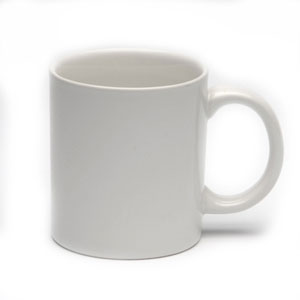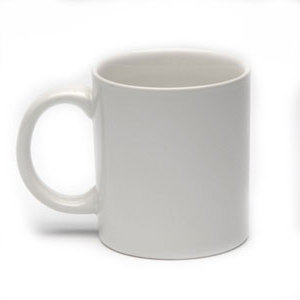Arguments: -m vertical -r 79 -l 120 -w 90 -p 10 -n 96 -e 2 -a -100 -v background -b none -f none -o -22+15 Arguments: -m vertical -r 73 -l 120 -w 90 -p 5 -n 94 -e 2 -a 100 -v background -b none -f none -o +20+15Overlay Example 3 Input Image (Source Image)Background Image (Source Image) Background Image (Source Image)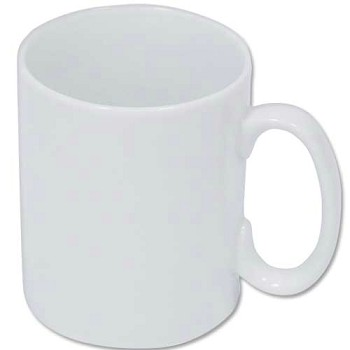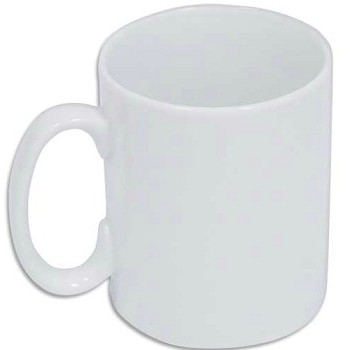Arguments: -m vertical -r 117 -l 150 -w 80 -p 17 -n 92 -e 2 \ -a -90 -v background -b none -f none -o -42+35 Arguments: -m vertical -r 117 -l 150 -w 80 -p 17 -n 94 -e 2 \ -a 90 -v background -b none -f none -o +39+35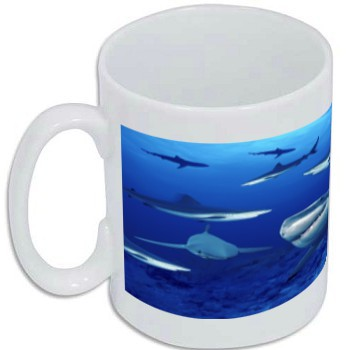Overlay Example 4 (image width < radius) Input Image (width=50, height=64)Input Image Padded From Width=50 To Width=74 (convert cartoon_cat.jpg -bordercolor none -border 12 cartoon_cat_pad.png)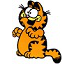Background Image (Source Image)Arguments: -m vertical -r 73 -l 64 -w 13 -p 5 -n 94 -e 2 -a 0 -v background -b none -f none -o +24+10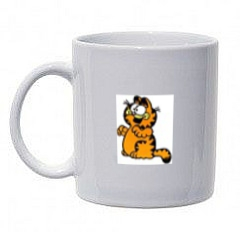Pitch in Both Directions Input Image (Source Image)Background ImageArguments: -m vertical -r 150 -l 310 -w 100 -p 6 -d both -e 1.4 -a 0 -v background -b none -f noneShading Input Image (Source Image)Background Image (Source Image)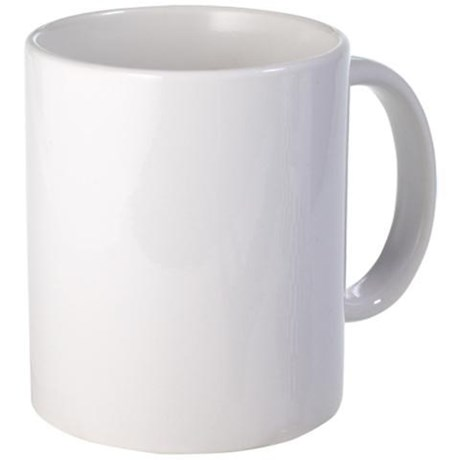Arguments: -m vertical -r 173 -l 290 -w 90 -p 12 -n 96 -e 2 -a -100 -v background -b none -f none -o -34+15 -c overArguments: -m vertical -r 173 -l 290 -w 90 -p 12 -n 96 -e 2 -a -100 -v background -b none -f none -o -34+15 -c multiply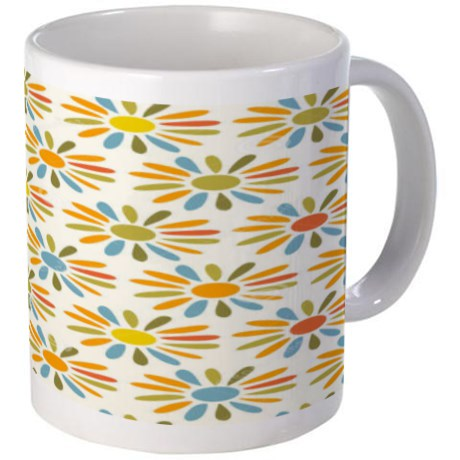What the script does is as follows: Computes an arcsin distortion map for the radial dimension Computes a linear distortion map for the length dimension Uses composite displace to apply the two distortion maps to the image This is equivalent to the following IM commands for the case of the default vertically oriented cylinder. width=`identify -ping -format %w \$infile` height=`identify -ping -format %h \$infile` xc=`convert xc: -format "%[fx:(\$width-1)/2]" info:` factor=`convert xc: -format "%[fx:2/pi]" info:` radius=`convert xc: -format "%[fx:\$width/4]" info:` length1=`convert xc: -format "%[fx:\$height]" info:` length1=`convert xc: -format "%[fx:\$length1*cos(pi*\$pitch/180)]" info:` height1=`convert xc: -format "%[fx:\$length1+\$radius1]" info:` ffx="ffx=\$factor*asin(xd);" convert -size \${width}x1 xc: -virtual-pixel black -fx \ "xd=(i-\$xc)/\$radius; \$ffx xs=0.5*(ffx+(\$xc-i)/(\$xc))+0.5; xd>1?1:xs" \ -scale \${width}x\${height1}! \ \$tmp0 ffx="ffx=-sqrt(1-(xd)^2);" convert -size \${width}x1 xc: -virtual-pixel black -fx \ "xd=(i-\$xc)/\$radius; \$ffx xs=0.5*(ffx)+0.5; abs(xd)>1?0.5:xs" \ -scale \${width}x\${height1}! \ \$tmp1 length2=`convert xc: -format "%[fx:100*(\$length1)/\$height]" info:` convert \$infile -resize 100x\${length2}% \ -gravity north -extent \${width}x\${height1} \$tmpA composite \$tmp0 \$infile \$tmp1 -virtual-pixel black \ -displace \${xc}x\${radius1} \$tmpA